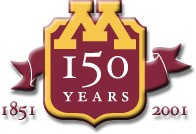# CSCI 5106: Programming Languages Using Scheme (Quick Guide)

Scheme is an interactive language. Typically, you create a Scheme program using some text editor you like and save it in a named file. I am going to assume that you already know how to use an editor such as emacs or vi; if you do not, you are very likely to find the course hard going and should rethink registering for it.

To use the Scheme system you may need to first load the module scheme. If you are new to the UMN setup and don't know about modules and how to load them, you can find some information about this in the system help pages; with specific regard to modules, you may look here.

To start up the Scheme system you simply type scheme at the shell command level. Once Scheme is up and running, it will prompt you for further input as exemplified by the following interaction:

```Scheme saved on Sunday November 21, 1993 at 9:15:23 PM
Release 7.3.1
Microcode 11.146
Runtime 14.166

1 ]=>
```
This signals the fact that Scheme is ready to calculate expressions that you type in. Perhaps the simplest kind of expression that you may type in is an arithmetic one. Scheme uses a prefix syntax, that is, one in which the operator is typed in first. An example interaction may thus be the following:
```1 ] => (+ 2 3)

;Value: 5

1 ] =>
```
What has happened here is that the user has typed in the expression (+ 2 3), Scheme has calculated the value of the expression as 5 and displayed this and it has then become ready for another expression to be input. This kind of interaction can be repeated ad infinitum. This is what is referred to as the Read-Eval-Print (REP) loop of Scheme; Scheme reads in an expression, evaluates it, prints the value and is ready for the next expression.

One of the kinds of expressions you can evaluate is that of loading the definitions of functions from a file. We will talk a little in class about function definitions in a language such as Scheme and also of the way lists are represented in it. You may also want to start looking at the MIT Scheme Reference Manual and you might try to write a few function definitions for yourself. A particular example of such a definition is the following one for appending two lists, i.e. for combining two lists into one.

```(define (append l1 l2)
(if (null? l1)
l2
(cons (car l1) (append (cdr l1) l2))))
```
The function that is defined by this code is append; make sure you understand how this works. Suppose, now, that this definition is stored in a file called app.scm relative to the current path. You can load this definition into Scheme by using a special load function. In particular, you would do the following at the Scheme prompt:
```1 ]=> (load "app.scm")
```
In detail, the load function takes a string (that is enclosed in Scheme within double quotes) as argument. This string is the name of a file. Scheme evaluates the expression above by looking at the code in the file and checking if they correspond to correct programs. In case they do not, Scheme indicates the errors in the code. On the other hand, if the definitions are correct, then Scheme compiles them and adds the functions to a list of those that you can use in forming new expressions.

In the case above, the definition in the file is correct. Loading will therefore be performed successfully and Scheme will be ready to use the function append in further expressions to be evaluated. Thus, you may use this function to append two lists:

```1 ]=> (append '(1 2 3) '(4 5 6))

;Value 10: (1 2 3 4 5 6)

1 ]=>
```

You can go on to load more functions and evaluate other interesting expressions using them at this point. Think of some simple program you may have written in some other language and spend some time trying to write this in Scheme instead and test out your thoughts.

When you are finished, you would want to quite the Scheme session. You can do this as follows:

```1 ]=> (exit)      ;; This quits the scheme REP loop

Kill Scheme (y or n)? y
```
At this point, you will be back at the command level.

Created by gopalan atsign cs dot umn dot edu. Maintained by ngopalan atsign umn dot edu and evw atsign umn dot edu. Last modified: August 30, 2019.

The views and opinions expressed in this page are strictly those of the page author(s). The contents of this page have not been reviewed or approved by the University of Minnesota.